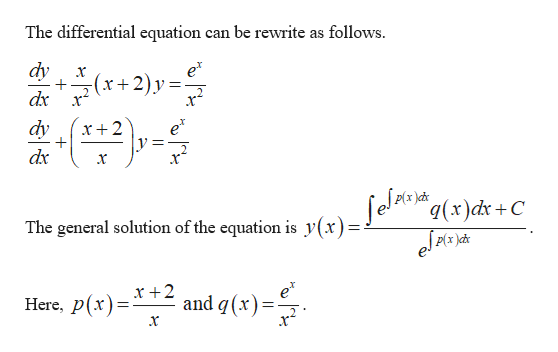Find the general solution of the given differential equation.x2(dy/dx) + x(x+2)y = exDetermine whether there are any transient terms in the general solution.

Question

Find the general solution of the given differential equation.

x2(dy/dx) + x(x+2)y = ex

Determine whether there are any transient terms in the general solution.

Step 1

The differential equation is x2(dy/dx) + x...help_outlineImage TranscriptioncloseThe differential equation can be rewrite as follows. dy +(r2)y = dx e* 2. dy x2 dx q(x)dx +C The general solution of the equation is y(x) eJAc Here, p(rX +2 and q(x)= fullscreen

Want to see the full answer?

See Solution

Want to see this answer and more?

Our solutions are written by experts, many with advanced degrees, and available 24/7

See Solution
Tagged in

Math